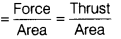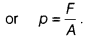# Define thrust and pressure. Give mathematical formula that relates thrust and pressure?

1. Define thrust and pressure.
2. Give mathematical formula that relates thrust and pressure.
1. Thrust is the force acting on a body perpendicular to its surface.
Thrust acting per unit area of the body is called pressure.
2. Mathematically, pressure